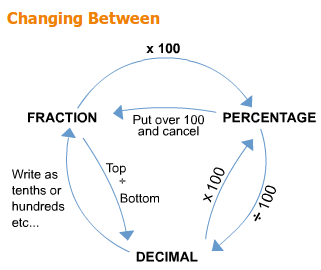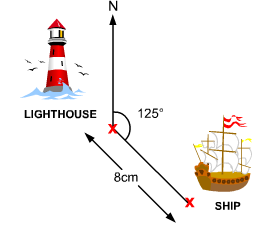# Percentages & Probability

• Created by: Kathryn
• Created on: 10-05-14 07:43

## Fractions

Always change mixed numbers to improper fractions before doing calculations!

Finding fractions of quantities - multiply by the top, divide by the bottom.

Multiplying

Multiply the tops (numerators) together and multiply the bottoms (denominators) together.

Dividing

Turn the second fraction upside-down and multiply as above.

Find the lowest common denominator. This is the lowest number that the denominators will both go into. Then multiply the top and bottom of each fraction by the same number (the number used for each fraction may be different!).

Now simply add (or subtract) the tops keeping the denominator the same!

1 of 11

## Ratios

Ratios behave like fractions. Find the total number of parts before dividing.

See what we mean with this one! Divide 35kg in the ratio 2:5. This means for every 2kg you put on the left put 5 on the right! 2 + 5 = 7 so we divide 35 by 7 giving 5. Now put 2 x 5 on the left and 5 x 5 on the right giving 10 : 25.2 of 11

## PercentagesFinding a percentage of a quantity:

1. Change the % to a decimal (divide by 100).
2. Multiply by the original quantity.

Increasing/decreasing a quantity by a percentage:

1. Find new percentage after increase/decrease (100 - % increase/decrease).
2. Change it to a decimal (divide by 100).
3. Multiply by the original quantity.

Compound interest:

1. Add interest on to 100%.
2. Change to a decimal (divide by 100).
3. Multiply the original amount by this decimal for each year.
3 of 11

## Simple Probability

1. We call 'something happening' an event. For example, getting a 6 with the roll of a dice.

2. Probability should always be written as a fraction, decimal or percentage, never '1 in 10' or '3 chances out of 5'.

3. The probability of something happening must be between 0 and 1 (unless you are using percentage - 0 to 100).

4. The sum of the probabilities of every possible outcome is 1.

5. The probability of something not happening is 1 minus the probability of it happening.

If we call a particular event 'A' then:p (not A) = 1 - p (A)

4 of 11

## Experimental Probablility

If you can do something a certain number of times and record the results you can write down estimates for the probabilities of each outcome.

These estimates are also known as Relative Frequencies.

## The 'and' rule

If you want one outcome or another outcome then you add their probabilities together.

For example:

For two events A and B, p (A or B) = p (A) + p (B)

The two events must be 'mutually exclusive'

5 of 11

## Tree Diagrams6 of 11

## The 'or' Rule

If you want one outcome or another outcome then you add their probabilities together.

For example: For two events A and B, p (A or B) = p (A) + p (B)

The events must be mutually exclusive.

• Brackets
• Of
• Division
• Multiplication
• Subtraction

The way you can avoid any mistakes is by:

1. Using the Memory.

2. Using the Bracket buttons.

7 of 11

## Angles

Types of Angles What they do...

Round a point Add up to 360o

On a straight line Add up to 180o

Vertically Opposite Equal

In a triangle Add up to 180o

In a quadrilateral (4-sided polygon) Add up to 360o

Alternate (Z-angles) Equal

Corresponding (F-angles) Equal

Supplementary (C or U angles) Add up to 180o

8 of 11

## Bearings

• Always use three figures.
• Always draw North lines.
• Always measure from North being 0o.
• Always measure clockwise.
• Always put in pencil lines connecting the points and don't rub them out at the end.
• Always put your protractor on the place you're measuring from and not to.
• Be as accurate as your can (sharp pencil, lean over diagram!).9 of 11

## Sequences

nth term = dn + (a - d)  where d is the difference and a is the first term.

If the second difference is constant (and first difference changes),where a is the first term, d is the difference between the first and second terms and c is the second difference.

Alternatively:

1. If the first difference keeps changing but the second difference is constant then the formula is something to do with 'n2'. Make a table showing the first few terms of 'n2'.
2. In the next column of your table write the differences between the term of 'n2' and your sequence.
3. Find the formula for this new sequence using dn + (a - d)
4. Add it on to n2 to give you your final formula
10 of 11

## Well-known Sequences

Below are some of the most common sequences. It is useful to be familier with these and their formulae.

Even numbers 2, 4, 6, 8, 10, ... nth term = 2n

Odd Numbers 1, 3, 5, 7, 9, ... nth term = 2n - 1

Square Numbers 1, 4, 9, 16, 25, ... nth term = n2

Cube Numbers 1, 8, 27, 64, 125, ... nth term = n3

Triangle Numbers 1, 3, 6, 10, 15, ... nth term = ½ n (n + 1)

Prime Numbers 2, 3, 5, 7, 11, 13, etc. No known formula!

Fibonacci Numbers 1, 1, 2, 3, 5, 8, 13, ... Formula not needed for GCSE. Each term is found by adding the previous term!

11 of 11